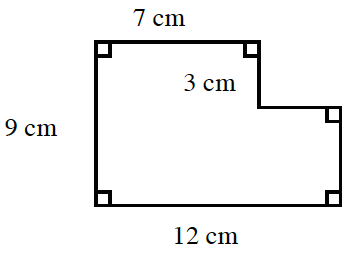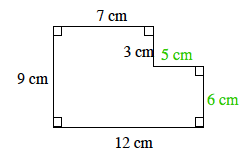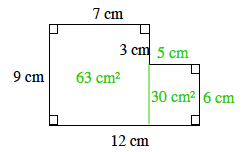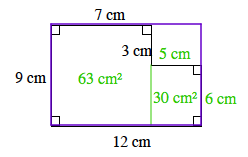### Home > ACC6 > Chapter 7 Unit 7 > Lesson CC1: 7.2.2 > Problem7-54

7-54.

Find the area of the shape at right in at least two ways.Label each remaining side using centimeters. An enclosed figure: Starting at the upper left corner: right 7, down 3, right 5, down 6, left 12, and up 9, to enclose the figure.

Our first method of finding the area is to divide the shape into smaller rectangles and label their areas. The enclosed figure is cut into two rectangles, by extending the 3 cm line down, to the 12 cm line. The rectangle on the left, is labeled 63 square centimeters, in its interior. The rectangle on the right, is labeled 30 square centimeters, in its interior.

$(9)(7) + (5)(6) = 63 + 30 \\= 93 \text{ square centimeters}$

Another method for finding the area is to subtract the area of the rectangle in the upper-right corner from the area of the purple rectangle. Show that this method gives the same answer as Method 1. The same figure with the top and right sides extended to have a 9 cm by 12 cm rectangle.